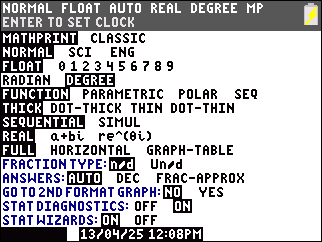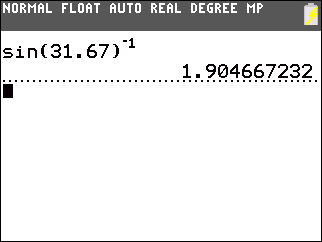# Knowledge Base

## Solution 34752: Calculating Secant, Cosecant, and Cotangent Using the TI-83 Plus and TI-84 Plus Family of Graphing Calculators.

### How do I calculate secant, cosecant and cotangent on the TI-83 Plus and TI-84 Plus Family of graphing calculators?

The TI-83 Plus and TI-84 Plus family of graphing calculators do not have built-in secant, cosecant and cotangent trigonometric functions. To compute these trigonometric functions, use the properties of trigonometric functions which are listed below.

cosecant(x) = csc(x) = 1/sin(x) = sin(x)-1

secant(x) = sec(x) = 1/cos(x) = cos(x)-1

cotangent(x) = cot(x) = 1/tan(x) = tan(x)-1

For example: Solve for the cosecant of 31.67 degrees.

• Be sure the calculator is set to Degree mode before beginning. To do this, press the [MODE] key, move the cursor to DEGREE and press [ENTER].